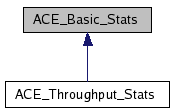# ACE_Basic_Stats Class Reference

Collect basic stats about a series of samples. More...

`#include <Basic_Stats.h>`

Inheritance diagram for ACE_Basic_Stats:[legend]
List of all members.

## Public Member Functions

ACE_Basic_Stats (void)
Constructor.
ACE_UINT32 samples_count (void) const
The number of samples received so far.
void sample (ACE_UINT64 value)
Record one sample.
void accumulate (const ACE_Basic_Stats &rhs)
Update the values to reflect the stats in rhs.
void dump_results (const ACE_TCHAR *msg, ACE_UINT32 scale_factor) const
Dump all the samples.

## Public Attributes

ACE_UINT32 samples_count_
The number of samples.
ACE_UINT64 min_
The minimum value.
ACE_UINT32 min_at_
The number of the sample that had the minimum value.
ACE_UINT64 max_
The maximum value.
ACE_UINT32 max_at_
The number of the sample that had the maximum value.
ACE_UINT64 sum_
The sum of all the values.

## Detailed Description

Collect basic stats about a series of samples.

Compute the average and standard deviation (aka jitter) for an arbitrary number of samples, using constant space. Normally used for latency statistics.

## Constructor & Destructor Documentation

 ACE_BEGIN_VERSIONED_NAMESPACE_DECL ACE_INLINE ACE_Basic_Stats::ACE_Basic_Stats ( void )

Constructor.

The number of samples is pre-allocated, and cannot changes once the class is initialized.

## Member Function Documentation

 ACE_BEGIN_VERSIONED_NAMESPACE_DECL void ACE_Basic_Stats::accumulate ( const ACE_Basic_Stats & rhs )

Update the values to reflect the stats in rhs.

 void ACE_Basic_Stats::dump_results ( const ACE_TCHAR * msg, ACE_UINT32 scale_factor ) const

Dump all the samples.

Prints out the results, using msg as a prefix for each message and scaling all the numbers by scale_factor. The latter is useful because high resolution timer samples are acquired in clock ticks, but often presented in microseconds.

 ACE_INLINE void ACE_Basic_Stats::sample ( ACE_UINT64 value )

Record one sample.

 ACE_INLINE ACE_UINT32 ACE_Basic_Stats::samples_count ( void ) const

The number of samples received so far.

## Member Data Documentation

The maximum value.

 ACE_UINT32 ACE_Basic_Stats::max_at_

The number of the sample that had the maximum value.

The minimum value.

 ACE_UINT32 ACE_Basic_Stats::min_at_

The number of the sample that had the minimum value.

 ACE_UINT32 ACE_Basic_Stats::samples_count_

The number of samples.

The sum of all the values.

The documentation for this class was generated from the following files:
Generated on Tue Jan 30 13:41:08 2007 for ACE by1.4.7-1